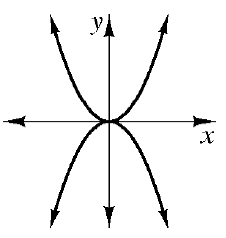### Home > INT2 > Chapter 9 > Lesson 9.2.2 > Problem9-66

9-66.

As you have seen, sometimes the graphs of two equations never intersect. However, when they do intersect, how many different intersection points can they have? How many intersection points can two lines have? How many intersections can two parabolas have? What about a parabola and a line? In this problem, you will investigate these questions. Sketch a graph that fits each description below. Not every graph is possible.

1. A line and a parabola that intersect twice.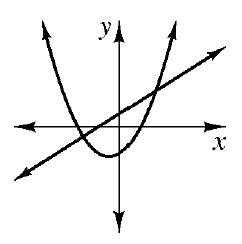2. A line and a parabola that only intersect once.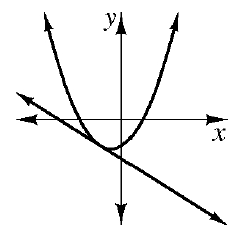3. A line and a parabola that intersect more than twice.

Can a straight line intersect a parabola more than twice?

4. Two parabolas that intersect twice.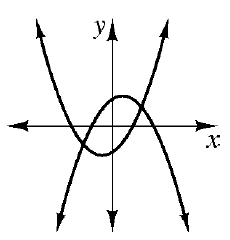5. Two parabolas that have an infinite number of intersections.

Can different parabolas intersect more than twice? How must two parabolas relate to each other so that they intersect each other an infinite amount of times?

6. Two parabolas that never intersect.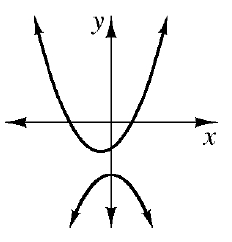7. Two parabolas that only intersect once.